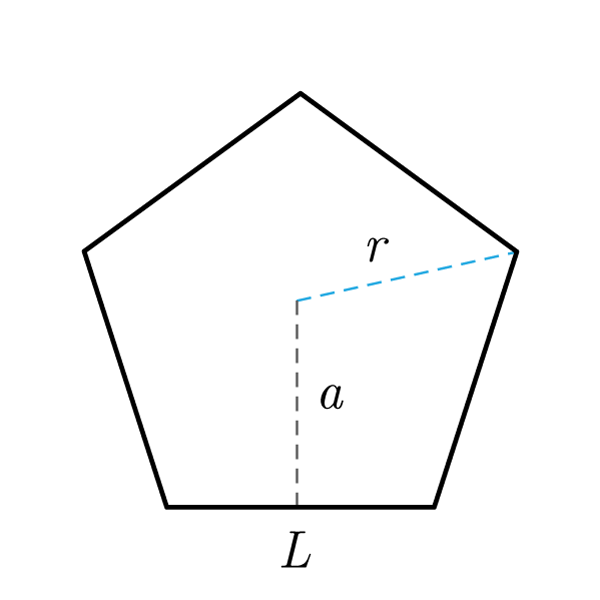# Pentagon

 Side $$S$$ Apothem $$a$$ Diagonal $$d$$ Circumcircle's Radius $$R$$
$$2p = S \times 5$$
Perimeter
$$S = \frac{2p}{5}$$
$$A = \frac{2p \times a}{2}$$
Area
$$a = \frac{2A}{2p}$$
Apothem
$$2p = \frac{2A}{a}$$
Perimeter
$$d = \frac{S (\sqrt{5} + 1)}{2}$$
Diagonal
$$S = \frac{d (\sqrt{5} - 1)}{2}$$
Side
Fixed number
$$f = 0.688 = \frac{a}{S}$$
Fixed number
$$a = S \times f$$
Apothem
$$S = \frac{a}{f}$$
Side
Area's costant
$$\varphi = 1.720 = \frac{A}{{S}^2}$$
Area's costant
$$A = {S}^2 \times \varphi$$
Area
$$S = \sqrt{\frac{A}{\varphi}}$$
Side
Inscribed Pentagon
$$S = \frac{ R\sqrt{10 - 2 \sqrt{5}} }{2}$$
Side
$$a = \frac{ R \times \left( \sqrt{5} + 1 \right) }{2}$$
Apothem
$$A = \frac{ {R}^2 \times 5 \sqrt{ 10 + 2 \sqrt{5} } }{8}$$
Area
$$R = d \left[ \sqrt{ \frac{5 - \sqrt{5}}{10} } \right]$$
$$d = R \left[ \sqrt{ \frac{5 + \sqrt{5}}{2} } \right]$$
Diagonal

## Definition

A pentagon is a polygon with five sides. A regular pentagon is a regular polygon with five sides and five angles congruent.

## Properties

1. Polygon with five sides
2. The regular pentagon has five sides and five angles congruent, with a measure of 108°
3. A regular pentagon can be inscribed into a circle or circumscribed by a circle## Pentagon Formulas

Data Formula
Perimeter 2p = S × 5
Area A = (2p × a) / 2
Diagonal d = [S(√5 + 1)] / 2
Side S = 2p / 5
Fixed number f = 0.688 = a / S
Area's costant φ = 1.720 = A / (S2)
Apothem a = (2A) / (2p)
Perimeter 2p = (2A) / (a)
Side S = [d(√5 - 1)] / 2
Apothem a = S × f
Side S = a / f
Area A = S2 × φ
Side S = √(A / φ)